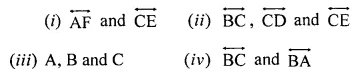ML Aggarwal Class 6 Solutions for ICSE Maths Model Question Paper 4 acts as the best resource during your learning and helps you score well in your exams.

## ML Aggarwal Class 6 Solutions for ICSE Maths Model Question Paper 4

Choose the correct answer from the given four options (1-2):
Question 1.
Avanti’s present age is y years and her mother’s age is 4 years less than 3 times her age, then her mother’s present age is
(a) (3y + 4) years
(b) (4y – 3) years
(c) (3y – 4) years
(d) 3(y – 4) years
Solution:Question 2.
Which of the following statements is false?
(a) Every square is a rhombus.
(b) An equilateral triangle is a regular polygon.
(c) A triangle having all acute angles is scalene.
(d) Every square is a regular polygon.
Solution: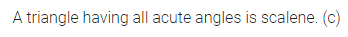Question 3.
If = 3, q = -2 and r = -1, find the value of: 2p2 + 3q – r2 + 2pr – 5pqr.
Solution:Question 4.
Fill in the following blanks:
(i) A polygon is a closed simple curve made up of entirely ………….
(ii) A cuboid has 6 rectangular faces, edges and ………. vertices.
Solution: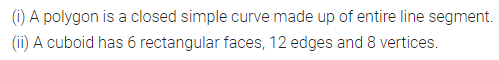Question 5.
Give reason(s) of using triangular shapes and not polygonal shapes consisting of four or more sides in constructing structures like electric towers and bridges. What value is added in using triangular shapes?
Solution:Question 6.
Look at the following pattern of squares fonned by matchsticks:Find the rule that gives the number of matchsticks required in terms of the number of squares formed.
Solution:Question 7.
Name each of the following triangles in two ways (you may judge by observation or use ruler and protractor):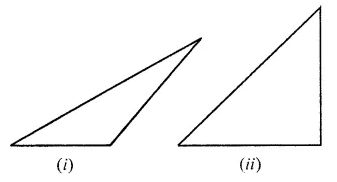Solution:Question 8.
Draw a net of a regular tetrahedron.
Solution:Question 9.
Solve the linear equation:
4 – 3(5x + 2) = 4(7 – 3x).
Also, verify the solution.
Solution: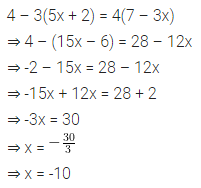Question 10.
Use the given figure to name:
(i) parallel lines
(ii) concurrent lines
(ii) collinear points
(iv) two opposite rays.Solution: